Economics, with Computers

Algorithmics, Econometrics, Data & Machine Learning

Arthur Charpentier - @freakonometrics - Univ. Rennes 1 & UQAM
2016

Freakonomics, with data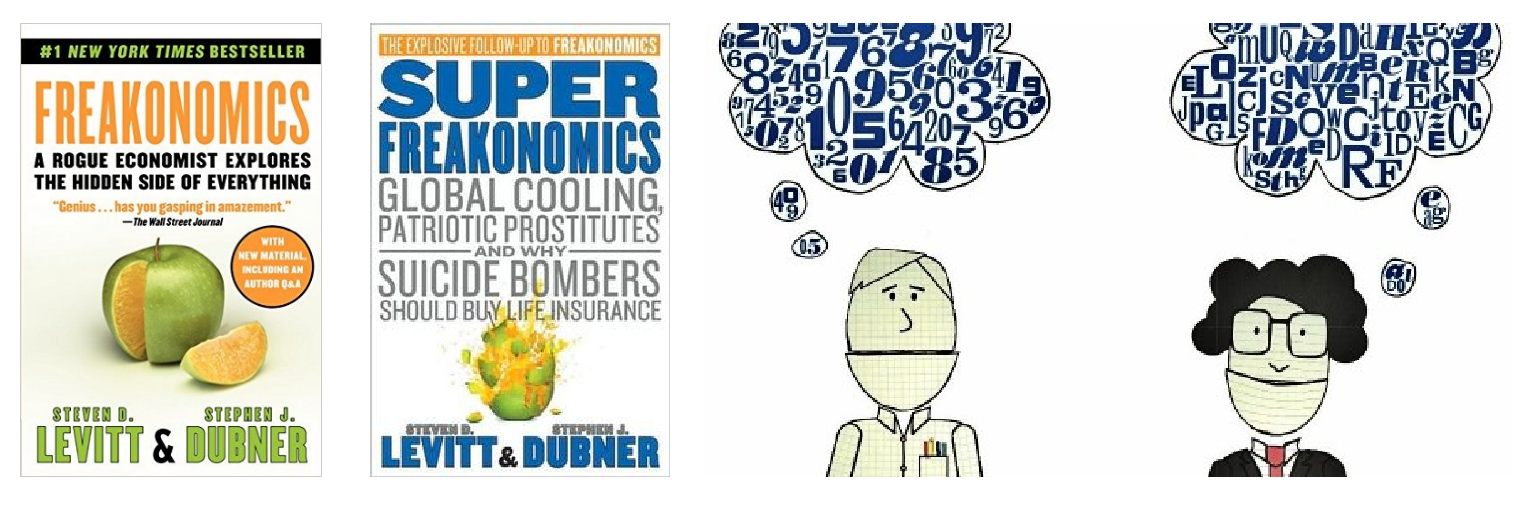Freakonomics: A Rogue Economist Explores the Hidden Side of Everything by Steven Levitt (Univ. of Chicago) & Stephen J. Dubner (New York Times).

A blog on freakonometrics ? (now called data science)freakonometrics.hypotheses.orgfreakonometrics

Data

"People use statistics as the drunken man uses lamp posts - for support rather than illumination" Andrew Lang (or not)Economics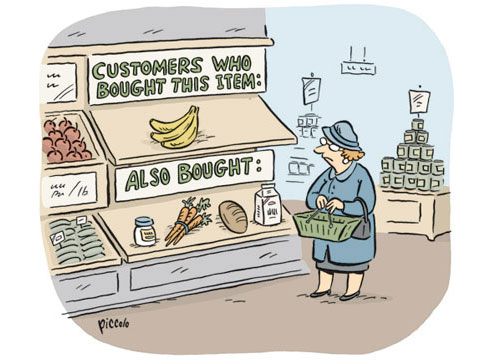Economics focuses on the behavior and interactions of economic agents and how economies work.

Economics & AlgorithmsSee Eisen (2011) Amazon's \$23,698,655.93 book about flies

Economics & Algorithms (HFT)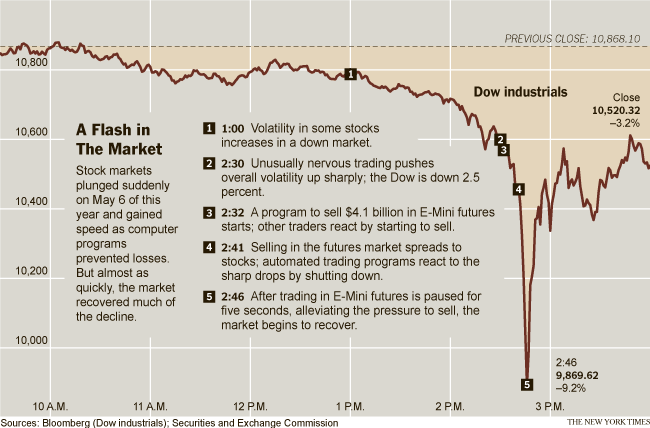See Wikipedia 2010 Flash Crash.

Computational Economics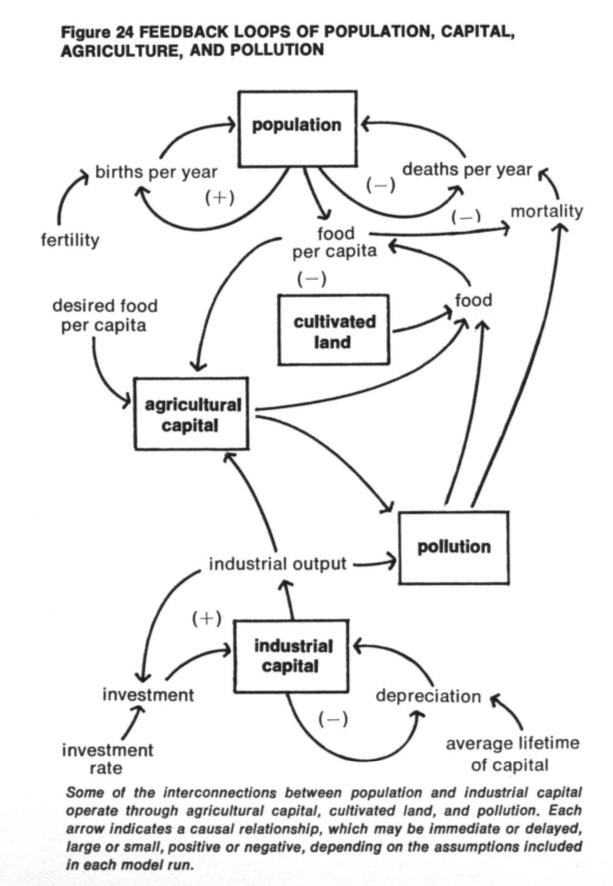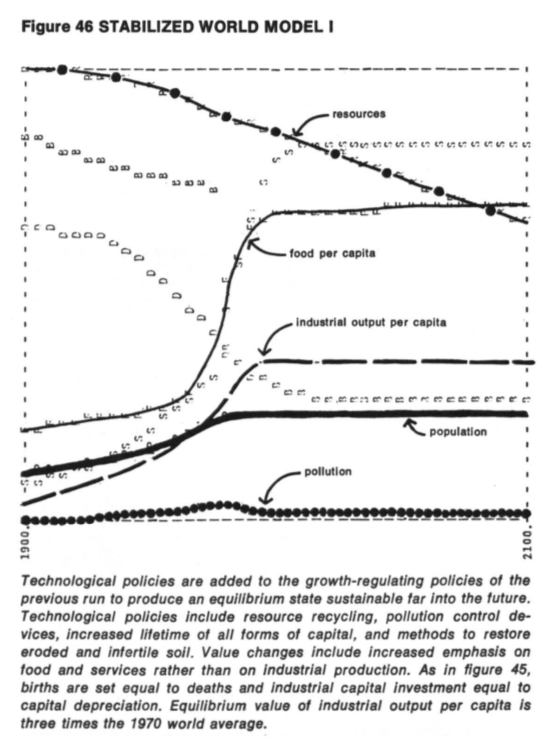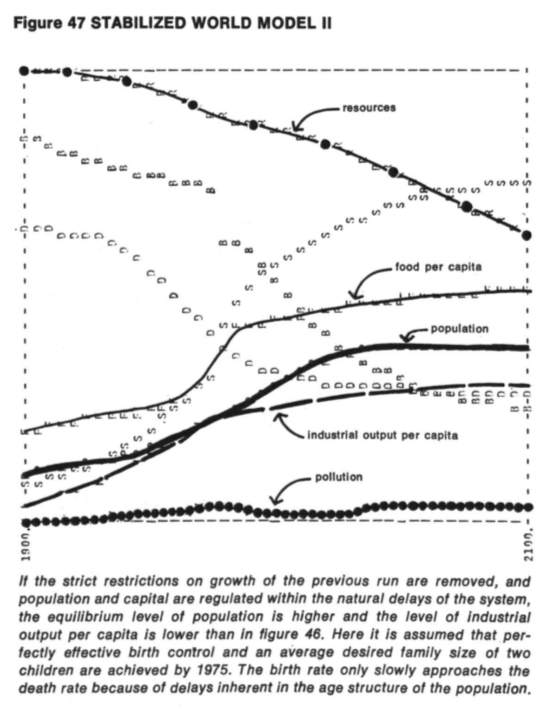Equilibrium of 'complex' dynamic systems, see Meadows et al. (1972) The Limits of Growth and Sargent & Stachurski (2015) on Quantitative Economics with Python

Econometrics: Economics & Data

"Econometrics is seens a vehicle for fundamental innovations in scientific method, above all, in the development of operative forecasting procedures in non-experimental situations"", see Wold (1969) Econometrics as Pioneering in Nonexperimental Model Building

Historically, econometric models were designed for macroeconomic data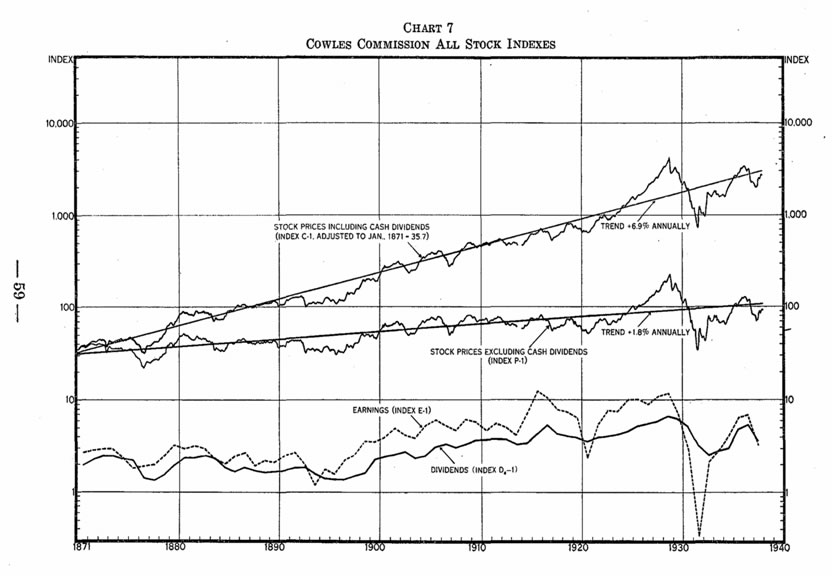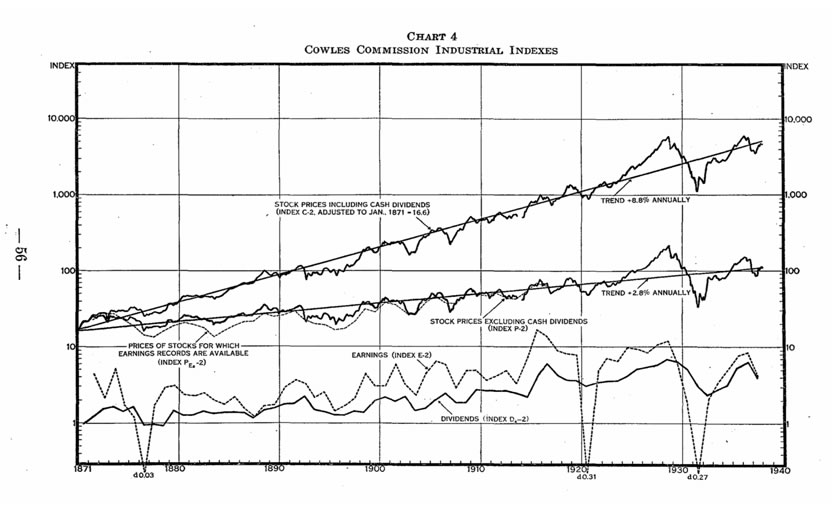Econometrics: Economics & Data

Nowadays, more and more econometric models designed for microeconomic data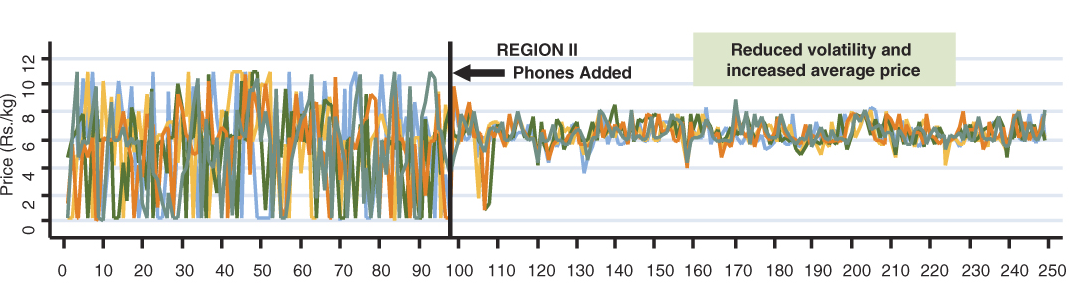See Jensen (2007) The Digital Provide: Information, Market Performance, and Welfare in the South Indian Fisheries Sector

Econometrics vs. Machine Learning

A supervised model for $y\in R~$ given $\boldsymbol{x}\in R^k~$ is a mapping $m:R^k\rightarrow R~$. A dataset is a collection of observations $(\boldsymbol{x}_i,y_i)~$, where $i=1,\cdots,n$.

In Econometrics, given some probability space $(\Omega,A,P)$, assume that $y_i$ are realization of i.i.d. variables $Y_i$ (given $\boldsymbol{X}_i=\boldsymbol{x}_i$) with distribution $F_i$. Then solve $\widehat{m}(\cdot)=\underset{m(\cdot)\in F}{\text{argmax}}\left\lbrace \log\mathcal{L}(m(\boldsymbol{x});\boldsymbol{y}) \right\rbrace = \underset{m(\cdot)\in F}{\text{argmax}}\left\lbrace \sum_{i=1}^n \log f(y_i; m(\boldsymbol{x}_i)) \right\rbrace$ where $\log\mathcal{L}$ denotes the log-likelihood.

In Machine Learning, given some dataset $(\boldsymbol{x}_i,y_i)$, solve $\widehat{m}(\cdot)=\underset{m(\cdot)\in F}{\text{argmin}}\left\lbrace \sum_{i=1}^n \ell(y_i,m(\boldsymbol{x}_i)) \right\rbrace$ for some loss functions $\ell(\cdot,\cdot)$. See Varian (2015) or Kaldero & Taurasi (2015)

Econometrics vs. Machine Learning

Consider an optimal smoothing problem: optimal $h$ for $\widehat{m}_h(\cdot)$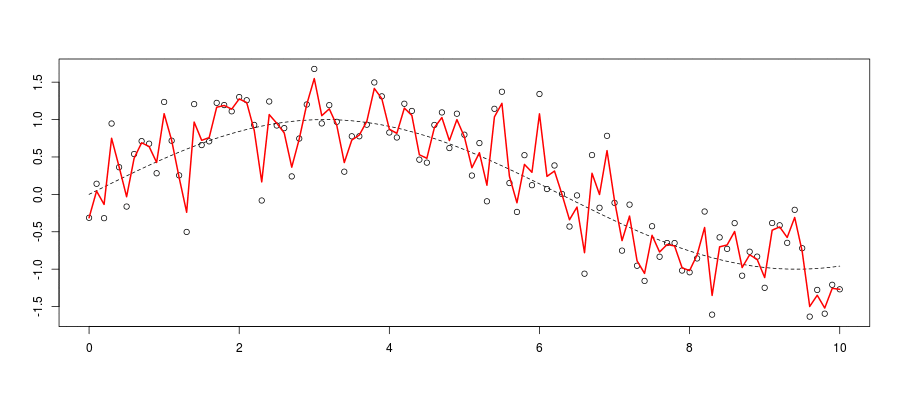Standard criteria, minimize the mean integrated squared error $\widehat{m}_{\color{red}{h}}(\cdot)$, $mise(\color{red}{h})=\mathbb{E}\left[\int\left( m(\boldsymbol{x})-\widehat{m}_{\color{red}{h}}(\boldsymbol{x}) \right)^2d\boldsymbol{x}\right]$

Econometrics vs. Machine Learning

In Econometrics, massive use of asymptotic statistical properties, i.e. CLT, $\sqrt{\color{blue}{n}}(\overline{X} - \mathbb{E}[X])\ \overset{L}\rightarrow N(0,\;\text{Var}[X])$ and Taylor expansion (delta method).

For some kernel based smoother, as $\color{blue}{n}\rightarrow\infty$ $mise(\color{red}{h}) \sim \frac{\color{red}{h}^4}{4}\left(\int x^2K(\boldsymbol{x})d\boldsymbol{x} \right)^2\int\left(m''(\boldsymbol{x})+2m'(\boldsymbol{x})\frac{f'(\boldsymbol{x})}{f(\boldsymbol{x})}\right)d\boldsymbol{x} \\ +\frac{1}{\color{blue}{n}\color{red}{h}}\sigma^2\int K^2(\boldsymbol{x})d\boldsymbol{x} \int\frac{d\boldsymbol{x}}{f(\boldsymbol{x})}$ Thus, standard rule of thumb, $\color{red}{h^\star \sim \color{blue}{n}^{-\frac{1}{5}}}$ (up to some multiplicative constant).

Econometrics vs. Machine Learning

In Machine Learning, use bootstrap / cross validation.

E.g. for leave-on-out cross validation define $\widehat{mise}({\color{red}{h}})=\frac{1}{n}\sum [y_i - \widehat{m}_{\color{red}{h},(i)}(\boldsymbol{x}_i)]^2$ then set $\color{red}{h^\star}=\text{argmin}\lbrace\widehat{mise}({\color{red}{h}})\rbrace$

Machine learning methods are about algorithms, more than asymptotic statistical properties.

Economics in 1965-1985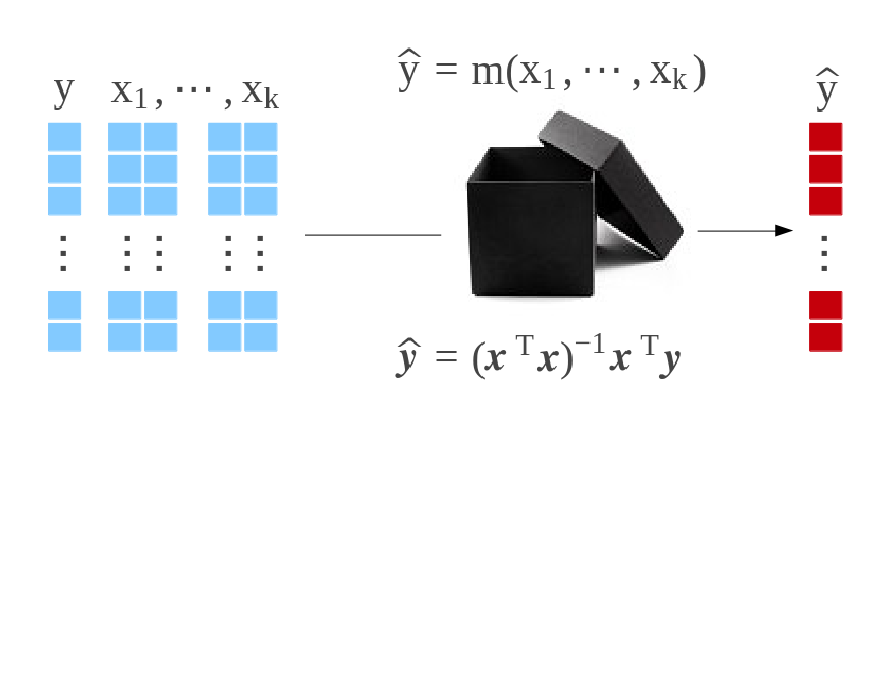Economics in 2015-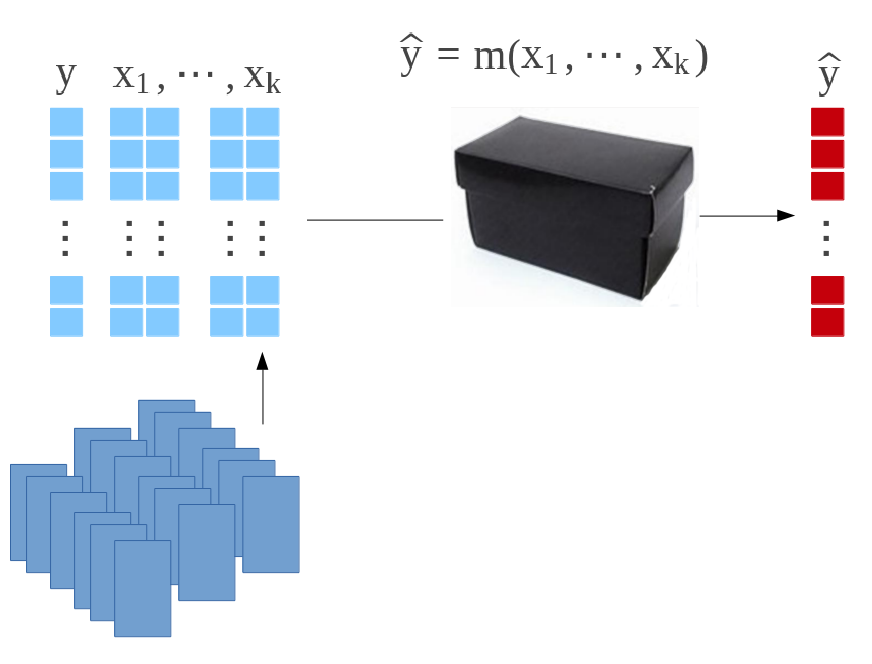Machine Learning & AIMachine Learning & AI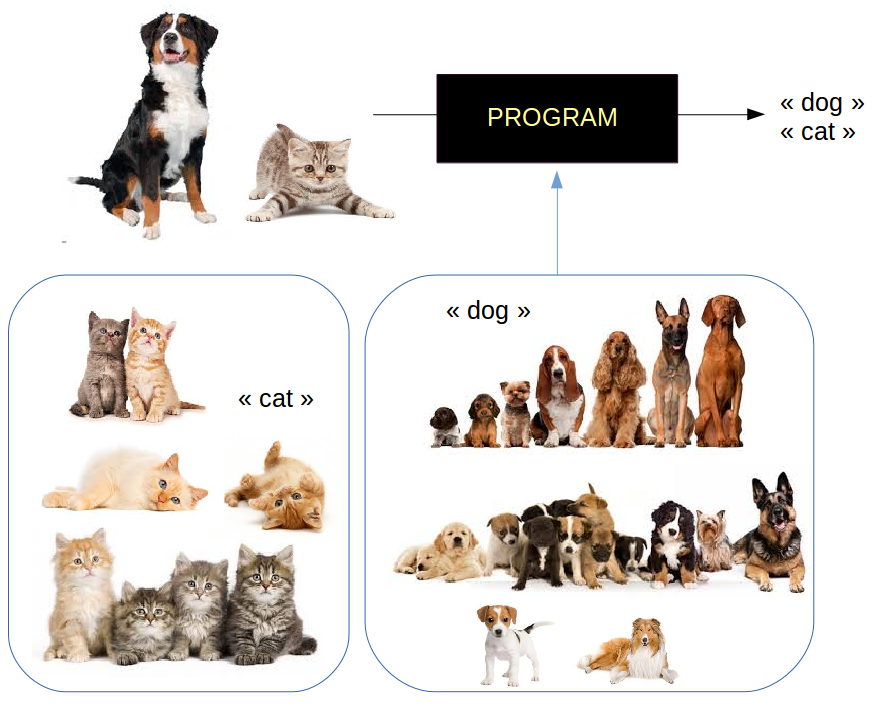Big or Small Data

Big data, scalability issues but good news to test microeconomic models, e.g. oil price, prixdescarburants.info and data.gouv.fr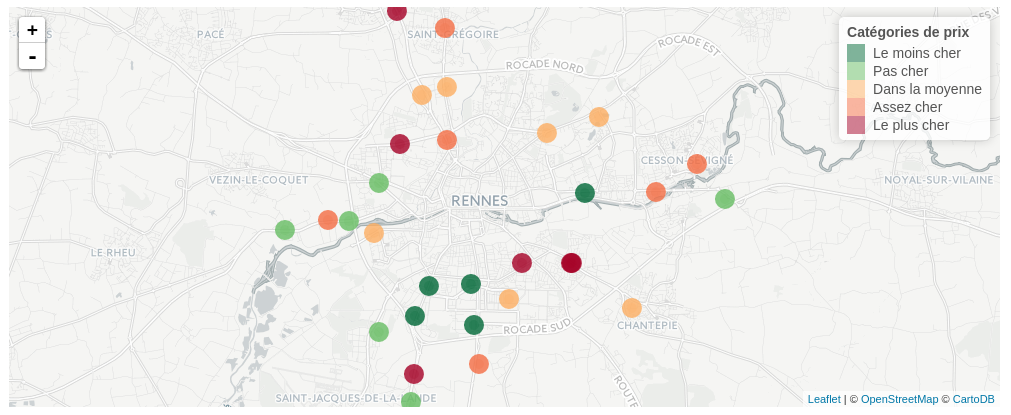Small data,

• sampling techniques (e.g. selection bias)

• aggregation techniques (e.g. ecological fallacy)

Ecological Fallacy

E.g. Simpson's Paradox, Blyth (1972), or Charpentier (2015)

Hospital Admission Survival Death Survival Rate
A 1000 800 200 80%
B 1000 900 100 90%

For healthy people

Hospital Admission Survival Death Survival Rate
A 600 590 10 98%
B 900 870 30 97%

while for sick people

Hospital Admission Survival Death Survival Rate
A 400 210 190 53%
B 100 30 70 30%